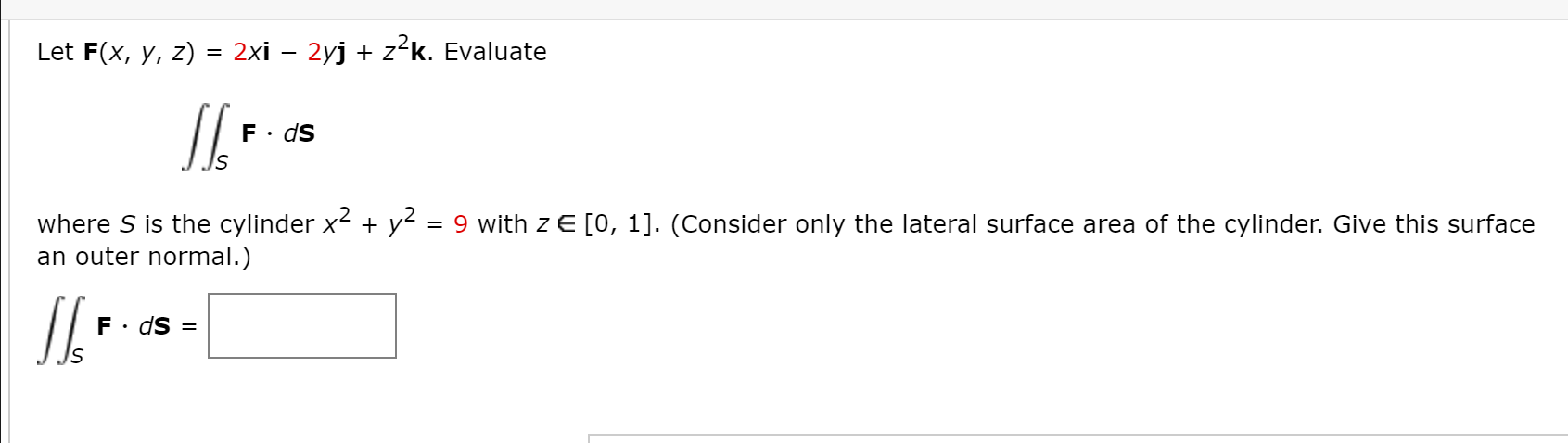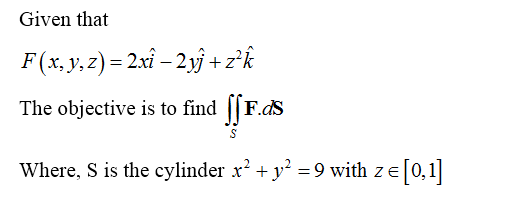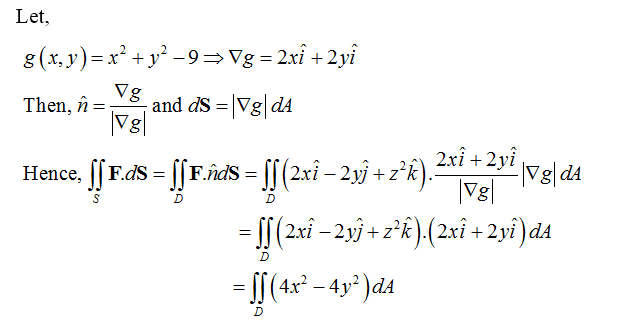# Let F(x, y, z) = 2xi – 2yj + z²k. EvaluateIswhere S is the cylinder x2 + y2 = 9 with z E [0, 1]. (Consider only the lateral surface area of the cylinder. Give this surfacean outer normal.)F· dS =

Question
4 viewshelp_outlineImage TranscriptioncloseLet F(x, y, z) = 2xi – 2yj + z²k. Evaluate Is where S is the cylinder x2 + y2 = 9 with z E [0, 1]. (Consider only the lateral surface area of the cylinder. Give this surface an outer normal.) F· dS = fullscreen
check_circle

Step 1Step 2...

### Want to see the full answer?

See Solution

#### Want to see this answer and more?

Solutions are written by subject experts who are available 24/7. Questions are typically answered within 1 hour.*

See Solution
*Response times may vary by subject and question.
Tagged in